Affine space

over a fieldA set(the elements of which are called the points of the affine space) to which corresponds a vector space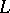over(which is called the space associated to) and a mapping of the setinto the space(the image of an element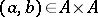is denoted by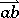and is called the vector with beginning in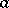and end in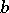), which has the following properties:

a) for any fixed pointthe mapping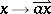,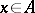, is a bijection ofon;

b) for any points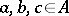the relationship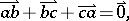wheredenotes the zero vector, is valid. The dimension ofis taken for the dimension of the affine space. A point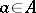and a vector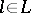define another point, which is denoted by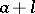, i.e. the additive group of vectors of the spaceacts freely and transitively on the affine space corresponding to.

Contents

Examples.

1) The set of the vectors of the spaceis the affine space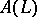; the space associated to it coincides with. In particular, the field of scalars is an affine space of dimension 1. If, then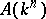is called the-dimensional affine space over the field, and its pointsanddetermine the vector.

2) The complement of any hyperplane in a projective space over the fieldis an affine space.

3) The set of solutions of a system of linear (algebraic or differential) equations is an affine space the associated space of which is the space of solutions of the corresponding homogeneous set of equations.

A subset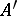of an affine spaceis called an affine subspace (or a linear manifold) inif the set of vectors,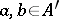, forms a subspace of. Each affine subspace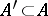has the form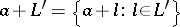, where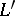is some subspace in, whileis an arbitrary element of.

A mapping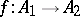between affine spacesandis called affine if there exists a linear mapping of the associated vector spacessuch that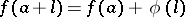for all,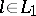. A bijective affine mapping is called an affine isomorphism. All affine spaces of the same dimension are mutually isomorphic.

The affine isomorphisms of an affine spaceinto itself form a group, called the affine group of the affine spaceand denoted by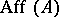. The affine group of the affine spaceis denoted by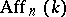. Each element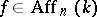is given by a formula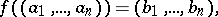where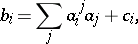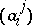being an invertible matrix. The affine groupcontains an invariant subgroup, called the subgroup of (parallel) translations, consisting of the mappings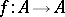for which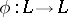is the identity. This group is isomorphic to the additive group of the vector space. The mappingdefines a surjective homomorphism ofinto the general linear group GL, with the subgroup of parallel translations as kernel. Ifis a Euclidean space, the pre-image of the orthogonal group is called the subgroup of Euclidean motions. The pre-image of the special linear group SGL is called the equi-affine subgroup (cf. Affine unimodular group). The subgroupconsisting of the mappingssuch that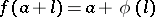for a givenand arbitraryis called the centro-affine subgroup; it is isomorphic to the general linear group GL of the space.

In algebraic geometry an affine algebraic set is sometimes called an affine space. A finite-dimensional affine space can be provided with the structure of an affine variety with the Zariski topology (cf. also Affine scheme).

Affine spaces associated with a vector space over a skew-fieldare constructed in a similar manner.